# Introduction of Real Number

#### notes

In your earlier classes, you have learnt about the number line and how to represent various types of numbers on it (see Fig. 1.1).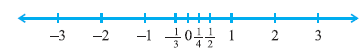Fig. 1.1 : The number line

Just imagine you start from zero and go on walking along this number line in the positive direction. As far as your eyes can see, there are numbers, numbers and numbers!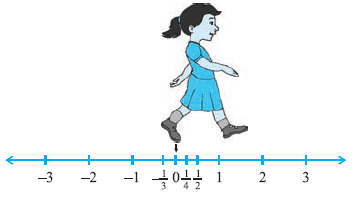Fig. 1.2

Now suppose you start walking along the number line, and collecting some of the numbers. Get a bag ready to store them!

You might begin with picking up only natural numbers like 1, 2, 3, and so on. You know that this list goes on for ever. Why is this true?) So, now your bag contains infinitely many natural numbers! Recall that we denote this collection by the symbol N.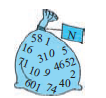Now turn and walk all the way back, pick up zero and put it into the bag. You now have the collection of whole numbers which is denoted by the symbol W.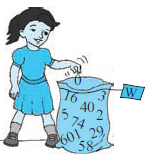Now, stretching in front of you are many, many negative integers. Put all the negative integers into your bag. What is your new collection? Recall that it is the collection of all integers, and it is denoted by the symbol Z.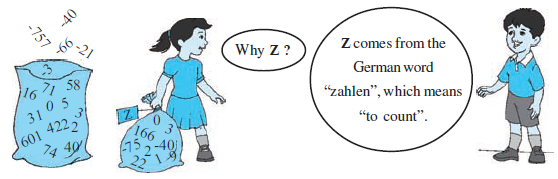Are there some numbers still left on the line? Of course! There are numbers like 1/2,3/4,  or even (-2005)/2006. If you put all such numbers also into the bag, it will now be the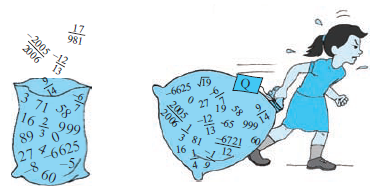collection of rational numbers. The collection of rational numbers is denoted by Q. ‘Rational’ comes from the word ‘ratio’, and Q comes from the word ‘quotient’. You may recall the definition of rational numbers:

A number ‘r’ is called a rational number, if it can be written in the formp/q, where p and q are integers and q ≠ 0. (Why do we insist that q ≠ 0?)

Notice that all the numbers now in the bag can be written in the formp/q,where p and q are integers and q ≠ 0. For example, –25 can be written as (-25)/1 ; here p = –25 and q = 1. Therefore, the rational numbers also include the natural numbers, whole numbers and integers. You also know that the rational numbers do not have a unique representation in the form p/q where p and q are integers and q ≠ 0. For example, 1/2=2/4=10/20=25/50=47/94,and so on. These are equivalent rational numbers (or fractions). However, when we say that p/q is a rational number, or when we represent p/q on the number line, we assume that q ¹ 0 and that p and q have no common factors other than 1 (that is, p and q are co-prime). So, on the number line, among the infinitely many fractions equivalent to 1/2, we will choose 1/2 to represent all of them.
Now, let us solve some examples about the different types of numbers, which you
have studied in earlier classes.
Example 1 : Are the following statements true or false? Give reasons for your answers.
(i) Every whole number is a natural number.
(ii) Every integer is a rational number.
(iii) Every rational number is an integer.
Solution : (i) False, because zero is a whole number but not a natural number.
(ii) True, because every integer m can be expressed in the form m/1, and so it is a rational number.
(iii) False, because 3/5 is not an integer.

Example 2 : Find five rational numbers between 1 and 2. We can approach this problem in at least two ways.
Solution 1 : Recall that to find a rational number between r and s, you can add r and s and divide the sum by 2, that is (r + s)/2 lies between r and s. So, 3/2 is a number between 1 and 2. You can proceed in this manner to find four more rational numbers between 1 and 2. These four numbers are 5/4,11/8,13/8 and 7/4

Solution 2 : The other option is to find all the five rational numbers in one step. Since we want five numbers, we write 1 and 2 as rational numbers with denominator 5 + 1, i.e., 1 =6/6 and 2 =12/6. Then you can check that 7/6,8/6,9/6,10/6 and 11/6 are all rational numbers between 1 and 2. So, the five numbers are 7/6, 4/3, 3/2, 5/3 and 11/6.

Remark : Notice that in Example 2, you were asked to find five rational numbers between 1 and 2. But, you must have realised that in fact there are infinitely many rational numbers between 1 and 2. In general, there are infinitely many rational numbers between any two given rational numbers.
Let us take a look at the number line again. Have you picked up all the numbers? Not, yet. The fact is that there are infinitely many more numbers left on the number line! There are gaps in between the places of the numbers you picked up, and not just one or two but infinitely many. The amazing thing is that there are infinitely many
numbers lying between any two of these gaps too!

If you would like to contribute notes or other learning material, please submit them using the button below.